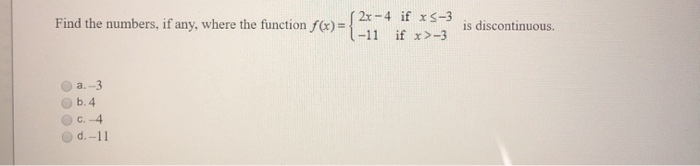# Question Solved1 AnswerFind the numbers, if any, where the function f(x) 2 (2x - 4 if xs-3 -11 if x>-3 is discontinuous 1 a.-3 O b.4 O c. 4 d.-11Transcribed Image Text: Find the numbers, if any, where the function f(x) 2 (2x - 4 if xs-3 -11 if x>-3 is discontinuous 1 a.-3 O b.4 O c. 4 d.-11
More
Transcribed Image Text: Find the numbers, if any, where the function f(x) 2 (2x - 4 if xs-3 -11 if x>-3 is discontinuous 1 a.-3 O b.4 O c. 4 d.-11